NCERT Exemplar: Areas Related to Circles - 1

# NCERT Exemplar: Areas Related to Circles - 1 - Notes | Study Mathematics (Maths) Class 10 - Class 10

 Table of contentsExercise 11.1Exercise 11.2Exercise 11.31 Crore+ students have signed up on EduRev. Have you?

Exercise 11.1

Choose the correct answer from the given four options:
Q.1. If the sum of the areas of two circles with radii R1 and R2 is equal to the area of a circle
(a)  R1 + R2 = R
(b)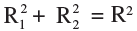(c)  R1 + R2 < R
(d)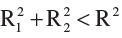Radius of first circle = R1
Area of first circle = πR12
Radius of second circle = R2
Area of second circle = πR22
Radius of third circle = R
Area of third circle = πR2
According to question
πR12 + πR22 = πR2
π(R12 + R22) =  πR2
R12 + R22 = R2
Hence option B is correct.

Q.2. If the sum of the circumferences of two circles with radii R1 and Ris equal to the circumference of a circle of radius R, then
(a)  R1 + R2 = R
(b) R1 + R2 > R
(c)  R1 + R2 < R
(d) Nothing definite can be said about the relation among R1, R2 and R.

According to the question,
Circumference of circle with radius R = Circumference of first circle with radius R1 + Circumference of second circle with radius R2
∴ 2πR = 2πR1+ 2πR2
⇒ R = R1+ R2
∴ Option A is correct.

Q.3. If the circumference of a circle and the perimeter of a square are equal, then
(a) Area of the circle = Area of the square
(b) Area of the circle > Area of the square
(c) Area of the circle < Area of the square
(d) Nothing definite can be said about the relation between the  areas of the circle and square.

Let the radius of the circle ber.
∴ Area of Circle = πr2 .
and the circumference = 2πr
The perimeter of the square is equal to circumference of circle.
∴ Its one side  = 1/4  × 2πr = πr/r.
So Area of Square = (πr/r)2.
∴ Area of Square/Area of circle  = πr2 :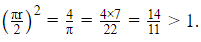So, Area of Circle > Area of Square.

Q.4. Area of the largest triangle that can be inscribed in a semi-circle of radius r units is
(a) r2sq. units
(b) 1/2 r2 sq. units
(c) 2 rsq. units
(d) √2 r2 sq. units

The largest triangle is isosceles triangle and Area = 1/2r2 × 2 = r2

Q.5. If the perimeter of a circle is equal to that of a square, then the ratio of their areas is
(a) 22 : 7
(b) 14 : 11
(c) 7 : 22
(d) 11: 14

Let radius of circle be r and side of a square be a.
Perimeter of a circle = Perimeter of a square
∴ 2πr = 4a
⇒ a = πr/2  .....(i)
Now, Area of circle/Area of square =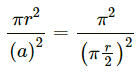......[From equation (i)]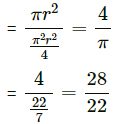= 14/11

Q.6. It is proposed to build a single circular park equal in area to the sum of areas of two circular parks of diameters 16 m and 12 m in a locality. The radius of the new park would be
(a) 10 m
(b) 15 m
(c) 20 m
(d) 24 m

Let the radius of new park be r.
∴πr2 = π(8)+ π(6)2
⇒ πr2 = 64π + 36π
⇒ πr2 = 100π
⇒ r = 10m

Q.7. The area of the circle that can be inscribed in a square of side 6 cm is
(a) 36 π cm2
(b) 18 π cm2
(c) 12 π cm2
(d) 9 π cm
2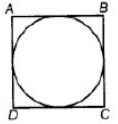Given, side of square = 6cm

∴ Diameter of a circle, (d) = side of square = 6cm
∴ Radius of a circle (r) = d/2 = 6/2 = 3cm
∴ Area of circle= π(r)2
= π(3)2 = 9πcm2

Q.8. The area of the square that can be inscribed in a circle of radius 8 cm is
(a) 256 cm2
(b) 128 cm2
(c) 64√2 cm2
(d)  64 cm
2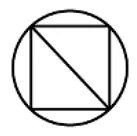Diagonal of square inscribed in a circle = Diameter of circle.
Therefore, d = 8 + 8 = 16cm
Side of square, a = d/ √2 = 16√2
Area of square =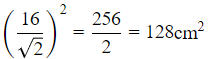Q.9. The radius of a circle whose circumference is equal to the sum of the circumferences of the two circles of diameters 36cm and 20 cm is
(a) 56 cm
(b) 42 cm
(c) 28 cm
(d) 16 cm

Diameter of first circle = d1 = 36 cm
Diameter of second circle = d2 = 20 cm
∴ Circumference of first circle = πd1 = 36π cm
Circumference of second circle = πd2 = 20π cm
Now, we are given that,
Circumference of circle = Circumference of first circle + Circumference of second circle
πD = πd1 + πd2
⇒ πD = 36π + 20π
⇒ πD = 56π
⇒ D = 56
⇒ Radius = 56/2 = 28 cm

Q.10. The diameter of a circle whose area is equal to the sum  of the areas of the two circles of radii 24 cm and 7 cm is
(a)  31 cm
(b) 25 cm
(c) 62 cm
(d) 50 cm

Area of first circle = πr2 = π(24)2 = 576π m2
Area of second circle = πr2 = π(7)2 = 49π m2
Now, we are given that,
Area of the circle = Area of first circle + Area of second circle
∴ πR2 = 576π +49π
(where, R is the radius of the new circle)
⇒ πR2 = 625π
⇒ R= 625
⇒ R = 25
∴ Radius of the circle = 25cm
Thus, diameter of the circle = 2R = 50 cm.

Exercise 11.2

Q.1. Is the area of the circle inscribed in a square of side a cm, πa2 cm2? Give reasons for your answer.

No, Let a be the side of square.
We are given that the circle is inscribed in the square.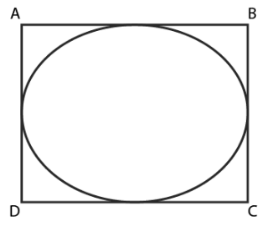Diameter of circle = Side of square = a
Radius of the circle = a/2
Area of the circle = πr2 = π(a/2)2 = (πa2)/4 cm2
Hence, area of the circle is (πa2)/4 cm2
Thus the area of the circle inscribed in a square of side a cm is not a2 cm2

Q.2. Will it be true to say that the perimeter of a square circumscribing a circle of radius a cm is 8a cm? Give reasons for your answer.

Yes, Let r be the radius of circle = a cm
∴ Diameter of the circle = d = 2 × Radius = 2a cm
As the circle is inscribed in the square, therefore,
Side of a square = Diameter of circle = 2a cm
Hence, Perimeter of a square = 4 × (side) = 4 × 2a = 8a cm

Q.3. In Fig 11.3, a square is inscribed in a circle of diameter d and another square is circumscribing the circle. Is the area of the outer square four times the area of the inner square? Give reasons for your answer.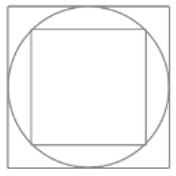Given diameter of circle is d.
∴ Diagonal of inner square = Diameter of circle = d
Let side of inner square EFGH be x.
∴ In right angled ΔEFG,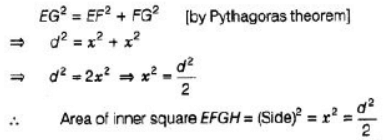But side of the outer square ABCS = Diameter of circle = d
∴ Area of outer square = d2
Hence, area of outer square is not equal to four times the area of the inner square.

Q.4. Is it true to say that area of a segment of a circle is less than the area of its corresponding sector? Why?

It is not true because in case of major segment, area is always greater than the area of its corresponding sector. It is true only in the case of minor segment.
Thus, we can conclude that it is not true to say that area of a segment of a circle is less than the area of its corresponding sector.

Q.5. Is it true that the distance travelled by a circular wheel of diameter d cm in one revolution is 2 π d cm? Why?

The given statement is is not true because the distance travelled by the wheel in one revolution is equal to its circumference.
⇒ Distance travelled = π d
⇒ Distance travelled = π (2 r) = 2πr [i.e. Circumference of wheel]

Q.6. In covering a distance s metres, a circular wheel of radius r metres makes S/2πr revolutions. Is this statement true? Why?

The distance travelled by a circular wheel of radius r m in one revolution is equal to the circumference of the circle = 2πr
No. of revolutions completed in 2πr m distance = 1
No. of revolutions completed in 1 m distance = (1/2πr)
No. of revolutions completed in s m distance = (1/2πr) × s = s/2πr
Thus, the statement “in covering a distance s metres, a circular wheel of radius r metres makes s/2πr revolutions” is true.

Q.7. The numerical value of the area of a circle is greater than the numerical value of its circumference. Is this statement true? Why?

If 0< r< 2, then numerical value of circumference is greater than numerical value of area of circle and if r > 2, area is greater than circumference.

Q.8. If the length of an arc of a circle of radius r is equal to that of an arc of a circle of radius 2 r, then the angle of the corresponding sector of the first circle is double the angle of the corresponding sector of the other circle. Is this statement false? Why?

Consider two circles C1  and C2  of radii r and 2r respectively.
Let the length of two arcs be l1  and l2.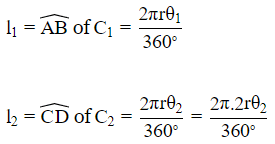According

l1  = l2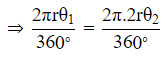⇒ θ1 = 2θ2
i.e., Angle of sector of the 1st circle is twice the angle of the sector of the other circle. Hence, the given statement is true.

Q.9. The areas of two sectors of two different circles with equal corresponding arc lengths are equal. Is this statement true? Why?

No, Area of first sector = (1/2)(r1)2θ1 ,
θ1 is the angle in radians subtended by the arc at the center of the circle.
Area of second sector = (1/2)(r2)2θ2,
θ2 is the angle in radians subtended by the arc at the center of the circle.
Given that: (1/2)(r1)2θ1 = (1/2)(r2)2θ2
⇒ (r1)2θ1 = (r2)2θ2
It depends on both radius and angle subtended at the center. But arc length only depends on radius of the circle. Therefore, it is not necessary that the corresponding arc lengths are equal. It is possible only if corresponding angles are equal (because then, the corresponding radii will be equal and hence the arc lengths will be equal).

Q.10. The areas of two sectors of two different circles are equal. Is it necessary that their corresponding arc lengths are equal? Why?

Yes, It is true for arcs of the same circle. But in different circle, it is not possible.

Q.11. Is the area of the largest circle that can be drawn inside a rectangle of length a cm and breadth b cm (a > b) is π b2 cm2? Why?

The largest circle that can be drawn inside a rectangle is possible when rectangle becomes a square.
∴ Diameter of the circle = Breadth of the rectangle = b
∴ Radius of the circle = b/2
Hence area of the circle = πr2 = π(b/2)2

Q.12. Circumferences of two circles are equal. Is it necessary that their areas be equal? Why?

True, If circumference of two circles are equal, then their corresponding radii are equal. So, their areas will be equal.

Q.13. Areas of two circles are equal. Is it necessary that their circumferences are equal? Why?

True, If areas of two circles are equal, then their corresponding radii are equal. So, their circumference will be equal.

Q.14. Is it true to say that area of a square inscribed in a circle of diameter p cm is p2 cm2? Why?

False, When the square is inscribed in the circle, the diameter of a circle is equal to the diagonal of a square but not the side of the square.
Let side of square = a
∴ p2 = a2 + a2

⇒ p2 = 2a2
⇒ a2 = p2/2
Hence area of square = a2 = p2/2

Exercise 11.3

Q.1. Find the radius of a circle whose circumference is equal to the sum of the circumferences of two circles of radii 15 cm and 18 cm.

Let the radius of a circle be r.
∴ Circumference of a circle = 2πr
Let the radii of two circles are r and r whose values are 15 cm and 18 cm respectively.
i.e. r1 = 15 cm and r2 = 18 cm
Now, by given condition,
Circumference of circle = Circumference of first circle + Circumference of second circle
⇒ 2πr = 2πr1 + 2πr2
⇒ r = r1 + r2
⇒ r = 15 + 18
∴ r = 33 cm
Hence, required radius of a circle is 33 cm.

Q.2. In Fig. 11.5, a square of diagonal 8 cm is inscribed in a circle. Find the area of the shaded region.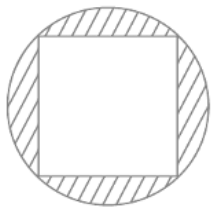Let the side of a square be a and the radius of circle be r.
Given that, length of diagonal of square = 8 cm
So, the area of the shaded region = Area of circle – Area of square
= (16π – 32) cm2
Hence, the required area of the shaded region is (16π – 32) cm2.

Q.3. Find the area of a sector of a  circle of radius 28 cm and central angle 45°.

Sector (OAPBO) of a circle is given whose
and central angle (θ) = 45
∴ Area of sector (A)= πr2θ /360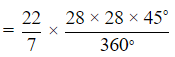⇒ Area of sector = 308 cm2 is the required area.

Q.4. The wheel of a motor cycle is of radius 35 cm. How many revolutions per minute must the wheel make so as to keep a speed of 66 km/h?

Radius of wheel = r = 35 cm
1 revolution of the wheel = Circumference of the wheel
= 2πr
= 2 × (22/7) × 35
= 220 cm
But, given that,
Speed of the wheel = 66 km/hr
= (66×1000×100)/60 cm/min
= 110000 cm/min
∴ Number of revolutions in 1 min = 110000/220 = 500
Hence, required number of revolutions per minute is 500.

Q.5. A cow is tied with a rope of length 14 m at the corner of a rectangular field of dimensions 20m × 16m. Find the area of the field in which the cow can graze.

Let ABCD be a rectangular field.
Length of field = 20 m
Breadth of the field = 16 m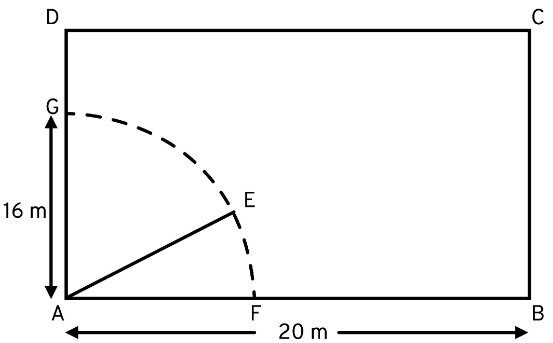According to the question,
A cow is tied at a point A.
Let length of rope be AE = 14 m = l.
Angle subtended at the center of the sector = 90°
Angle subtended at the center (in radians) θ = 90π/180 = π/2
∴ Area of a sector of a circle = ½ r2θ
= ½ × (14)× (π/2)
= 154 m2
Hence, the required area of a sector of a circle is 154 m2.

Q.6. Find the  area of the flower bed (with semi-circular ends) shown in Fig. 11.6.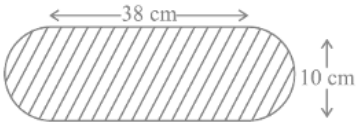Observing the figure, we get to know that It contains one rectangle of dimensions 38 cm × 10 cm and two semi-circles of diameters 10 cm. Area of Figure = Area of Rectangle + 2 × Area of Semicircle
Area of Rectangle = 38 × 10 = 380 cm2
Area of Semi-Circle =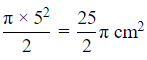Area of figure =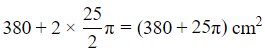Hence, option D is the correct answer.

Q.7. In Fig. 11.7, AB is a diameter of the circle, AC = 6 cm and BC = 8 cm. Find the area of the shaded region (Use π = 3.14).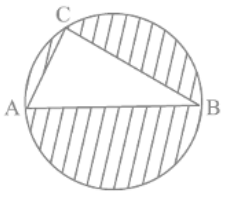Given, AB is the diameter of the circle.
The sides of triangle AC = 6 cm and BC = 8 cm
We have to find the area of the shaded region.
We know that a triangle in a semicircle is a right angled triangle with hypotenuse as diameter.
So, ACB is a right triangle with C at right angle
By pythagoras theorem,
AB² = AC² + BC²
AB² = (6)² + (8)²
AB² = 36 + 64
AB² = 100
Taking square root,
AB = 10 cm
Area of triangle = (1/2) × base × height
Area of triangle ABC = (1/2) × AC × BC
= (1/2) × 6 × 8
= 48/2
= 24 cm²
Diameter of circle = AB = 10 cm
Radius = 10/2 = 5 cm
Area of circle = πr²
= (3.14)(5)(5)
= (3.14)(25)
= 78.5 cm²
Area of shaded region = area of circle - area of triangle
= 78.5 - 24
= 54.5 cm²

Q.8. Find the area of the shaded field shown in Fig. 11.8.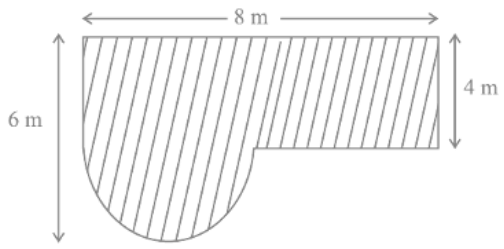In the figure, join ED.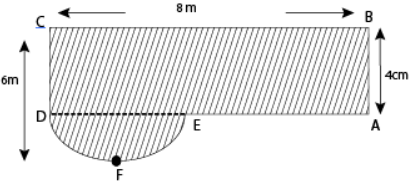From figure, radius of semi circle DFE , r = 6 – 4 = 2 m
Now, area of rectangle ABCD = BC × AB = 8 × 4 = 32 m2
Area of semi - circle DFE =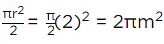∴ Area of shaded region = Area of rectangle ABCD + Area of semi-circle DFE= (32 + 2π)m2

Q.9. Find the area of the shaded region in Fig. 11.9.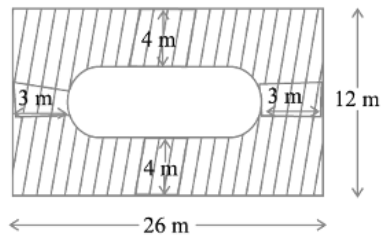Join GH and FE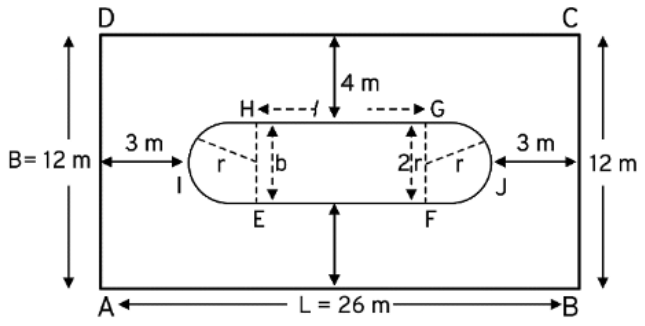Here, breadth of the rectangle BC = 12m∴ Breadth of the inner rectangle EFGH = 12 − (4 + 4) = 4cmwhich is equal to the diameter of the semi-circle EJF, d = 4m∴ Radius of semi-circle EJF , r = 2m∴ Length of inner rectangle EFGH = 26 − (5+5) = 16m
∴ Area of two semi-circles EJF and HIG =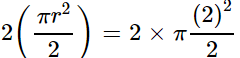= 4πm

Now, area of inner rectangle EFGH = EH × FG = 16 × 4 = 64m2

and area of outer rectangle ABCD = 26 × 12 = 312m2

∴∴ Area of shaded region = Area of outer rectangle - (Area of two semi - circles + Area of inner rectangle)

= 312 − (64 + 4π) = (248 = 4π)m2

Q.10. Find the area of the minor segment of a circle of radius 14 cm, when the angle of the corresponding sector is 60°.

Area of the minor segment is given by πr2 × θ/360o − r2 sin θ/2 cos θ/2
= πr2 × 60o/360o − r2 sin 30o
= 22/7 × 14 × 14 × 1/6 − 14 × 14 × 1/2 × √3/2
= (308/3 − 49√3)cm2

Q.11. Find the area of the shaded region in Fig. 11.10, where arcs drawn with centres A, B, C and D intersect in pairs at mid-points P, Q, R and  S of the sides AB, BC, CD and DA, respectively of a square ABCD (Use π = 3.14).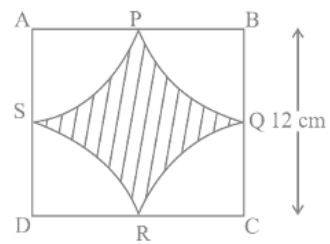Area of all four sectors made by square and circle are equal.
And each sector have an angle of 90°
And radius of circle is 12/2  cm = 6 cm
Area of shaded region = Area of square ABCD − 4 × Area of one sector
⇒ Area of shaded region = Area of square ABCD − Area of circle with radius 6 cm
= 12 × 12−π × 62 cm2
= 144−113.04 cm2
= 30.96 cm2

Q.12. In Fig. 1 1.11, arcs are drawn by taking vertices A, B and C of an equilateral triangle of side 10 cm. to intersect the sides BC, CA and AB at their respective mid-points D, E and F. Find the area of the shaded region (Use π = 3.14).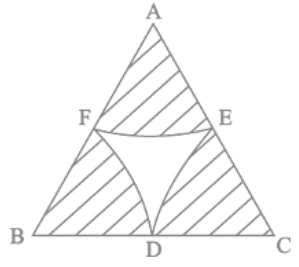From the given figure, area of the shaded part is equal to the sim of areas of these sectors at points A, B and C.
As ΔABC is equilateral triangle of side 10 cm and radius of the sectoris half of the side. All the three sectors are identical
θ = 60°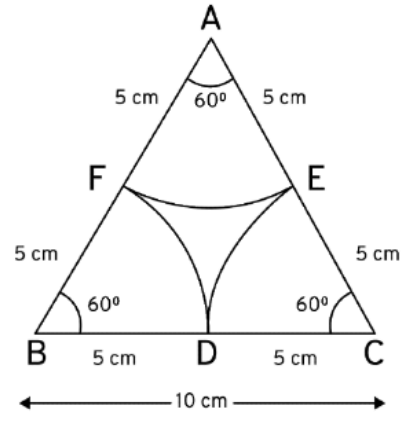Radius of each sector(r) = 10/2 = 5 cm
∴ Area of shaded part = 3 .(Area of sector)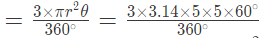=1.57 × 25 = 39.25 cm2

Q.13. In Fig. 11.12, arcs have been drawn with radii 14 cm each and with centres P, Q and R. Find the area of the shaded region.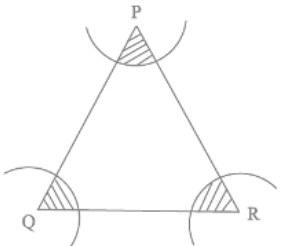The area of the shaded region is equal to the sum of areas of three sectors of same radius but of different angles θ1, θ2 and θ3.
∠θ1 + ∠θ2 + ∠θ3 = 180° [Int. ∠s of Δ]
∴ Area of shaded region =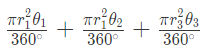where r1  = r2  = r3  = r = 14 cm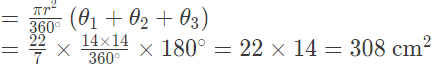∴ Area of shaded region = 308 cm2
Hence, required area = 308 cm2.

Q.14. A circular park is surrounded by a road 21 m wide. If the radius of the park is 105 m, find the area of the road.

Radius of the circular park, r = 105 m
Width of the road, d = 21 m
So, outer radius, r ′ = r + d = 126 m
Now, area of the road = area of the outer circle - area of the inner circle
= π × 1262 − π × 1052 = π(126− 1052)
= 22/7 × (126 + 105) × (126 − 105)
= 15246 m2

Q.15. In Fig. 11.13, arcs have been drawn of radius 21 cm each with vertices A, B, C and D of quadrilateral ABCD as centres. Find the area of the shaded region.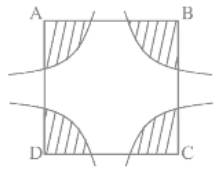Area of each sector is equal.

Area of shaded region =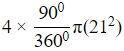= 22/7 × 212
= 1386 cm2

Q.16. A piece of wire 20 cm long is bent into the form of an arc of a circle subtending an angle of 60° at its centre. Find the radius of the circle.

Length of arc of circle = 20 cm
Here, central angle θ = 60
∴ Length of arc = θ/360 × 2πr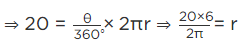r = 60/π cm
Hence, the radius of circle is 60/π cm

The document NCERT Exemplar: Areas Related to Circles - 1 - Notes | Study Mathematics (Maths) Class 10 - Class 10 is a part of the Class 10 Course Mathematics (Maths) Class 10.
All you need of Class 10 at this link: Class 10

## Mathematics (Maths) Class 10

53 videos|403 docs|138 tests
 Use Code STAYHOME200 and get INR 200 additional OFF

## Mathematics (Maths) Class 10

53 videos|403 docs|138 tests

Track your progress, build streaks, highlight & save important lessons and more!

,

,

,

,

,

,

,

,

,

,

,

,

,

,

,

,

,

,

,

,

,

;